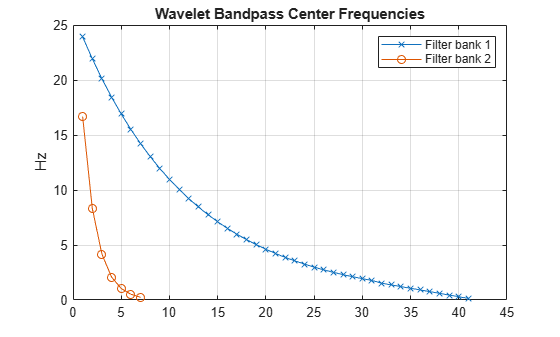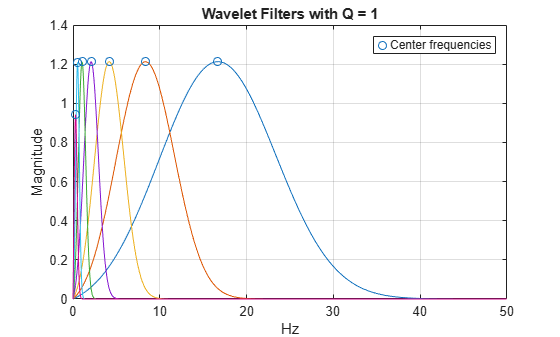# centerFrequencies

Wavelet scattering bandpass center frequencies

## Syntax

``F = centerFrequencies(sf)``
``F = centerFrequencies(sf,filterbanks)``

## Description

example

````F = centerFrequencies(sf)` returns the wavelet bandpass center frequencies for all filter banks of the wavelet time scattering network, `sf`. The output `F` is a cell array with Nfb elements, where Nfb is the number of scattering filter banks. Each element of `F` is a real-valued vector. If you specify a sampling frequency in `sf`, `F` is in hertz. If you do not specify a sampling frequency, `F` is in cycles/sample.If there is only one filter bank in the scattering network, `F` is a real-valued vector containing the wavelet bandpass center frequencies.```
````F = centerFrequencies(sf,filterbanks)` returns the wavelet bandpass center frequencies for the specified `filterbanks`. The argument `filterbanks` is a scalar or vector with all the elements between 1 and Nfb inclusive, where Nfb is the number of scattering filter banks.```

## Examples

collapse all

Create a wavelet time scattering network with a sampling frequency of 50 Hz.

`sf = waveletScattering('SamplingFrequency',50)`
```sf = waveletScattering with properties: SignalLength: 1024 InvarianceScale: 10.2400 QualityFactors: [8 1] Boundary: 'periodic' SamplingFrequency: 50 Precision: 'double' OversamplingFactor: 0 OptimizePath: 0 ```

Plot the wavelet bandpass center frequencies for all the filter banks.

```bpcf = centerFrequencies(sf); plot(bpcf{1},'rx-') hold on plot(bpcf{2},'bo-') grid on title('Wavelet Bandpass Center Frequencies') legend('Filter Bank 1','Filter Bank 2') ylabel('Hz')```Plot the wavelets filters used in computing the second-order scattering coefficients.

```orderCoef = 2; [filters,f] = filterbank(sf); figure plot(f,filters{orderCoef+1}.psift) grid on title('Wavelet Filters with Q = 1') xlabel('Hz') ylabel('Magnitude') hold on pl = plot(bpcf{orderCoef},max(filters{orderCoef+1}.psift),'bo'); legend(pl,'Center Frequencies')```.

## Input Arguments

collapse all

Wavelet time scattering network, specified as a `waveletScattering` object.

Filter bank indices, specified as a positive integer or vector of integers. Elements of `filterbanks` are integers between 1 and Nfb inclusive, where Nfb is the number of scattering filter banks.

Example: `F = centerFrequencies(sf,[1 2])` returns the wavelet bandpass center frequencies for the first two filter banks in `sf`.

Data Types: `double`

## Output Arguments

collapse all

Wavelet bandpass center frequencies for filter banks of the scattering network `sf`, returned as a vector or cell array of vectors. If there is only one filter bank in `sf`, or if `filterbanks` is a scalar, then `F` is a real-valued vector. Otherwise, `F` is a cell array, where each element is a real-valued vector.

Data Types: `double`

## Version History

Introduced in R2018b